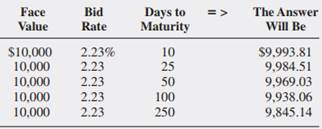### Create an Account

Home / Questions / Using a Spreadsheet to Calculate T bill Prices What is the bid price of a \$10000 face valu...

# Using a Spreadsheet to Calculate T bill Prices What is the bid price of a \$10000 face value T bill with a bid rate of 223 percent if there

Using a Spreadsheet to Calculate T-bill Prices: What is the bid price of a \$10,000 face value T-bill with a bid rate of 2.23 percent if there are 10, 25, 50, 100, and 250 days to maturity? (LG 5-2)Apr 26 2020 View more View LessSubscribe To Get Solution